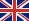# StarGazer - Astrological ConvertoCalculator (Galaxy.StarGazer)StarGazer - Astrological ConvertoCalculator (Galaxy.StarGazer) - this astrological calculator and converter allows you to perform various astrological calculations related to date-time, Julian day, degrees, coordinates, etc., as well as convert one view format to others. It will be useful both for astrologers-researchers, and aspiring astrologers who comprehend the basics of astrology. The program greatly simplifies data entry and eliminates the astrologer from mistakes, using maps already created in Galaxy programs. This program is free and is included in Galaxy astrological programs.

## Functionality of program

 Modes of operation Universal calculator; Arithmetic operations for calendar date-time; Arithmetic operations for the Julian Day; Arithmetic operations on magnitudes in degree measure; Arithmetic operations on the values in the format of degrees Zodiac; Local sidereal time calculator (LST); Zodiac converter degrees - absolute degrees; Gregorian date - Julian date converter; Converter calendar date-time - Julian Day; Geographic coordinate format converter; Degree format formats converter; Calendar date and time formats converter; Time measures format converter; Calculator of average values.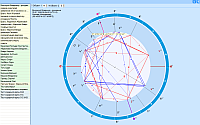The screen for entering values from the selected chart.Galaxy programs allow you to transfer a list of charts or individual charts through the clipboard to StarGazer, and an astrologer can select a specific chart from the transferred list and take one or another value from it depending on the calculation or conversion mode. Thus, the program minimizes manual input, which avoids human input errors.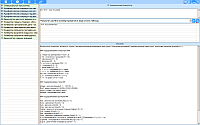Universal calculator.The calculator allows you to calculate a string with a mathematical expression in the form of numbers, logical expressions, arithmetic operators, trigonometric functions, etc.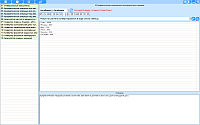Arithmetic operations for the calendar date-time.Arithmetic operations (addition, subtraction, multiplication and division by a constant) for the calendar date. Astrologers can enter values either manually or using the selected chart from the list.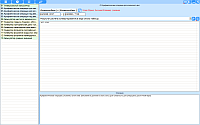Arithmetic operations for the calendar date-time.Arithmetic operations (addition, subtraction, multiplication and division by a constant) for the calendar date. Astrologers can enter values either manually or using the selected chart from the list.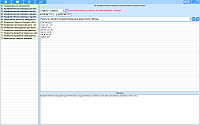Arithmetic operations on quantities in degree measure.Arithmetic operations on quantities in degree measure (addition, subtraction, multiplication and division by a constant).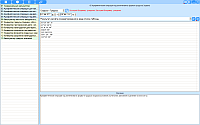Arithmetic operations on values in the format of degrees of the Zodiac.Arithmetic operations on values in the format of degrees of the zodiac (addition, subtraction, multiplication and division by a constant).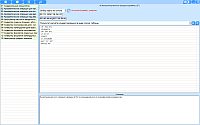Calculator of local sidereal time (LST).Calculate local sidereal time (LST) by calendar date and geographic coordinates.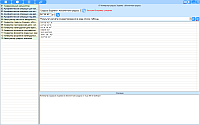Zodiac converter degrees are absolute degrees.Zodiac converter degrees to absolute degrees from 0 to 360 and vice versa.Gregorian Date Converter - Julian Date.Gregorian Date Converter to Julian and vice versa.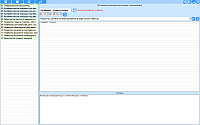The calendar date-time converter is a Julian day.The calendar date converter is a Julian day and vice versa.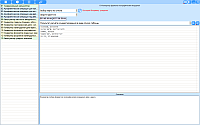Geographical coordinates format converter.Converter allows you to convert any geographic coordinate formats into each other. For example, you can easily convert the coordinates from Wikipedia into any format that astrological programs understand.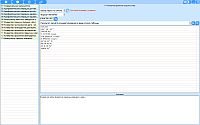Converter of formats of degree measures.Converter of any formats of degree measures of each other.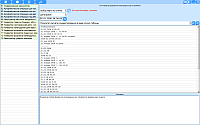Converter of formats of calendar dates and times.Converter of any formats of calendar dates and time formats into each other.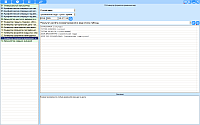Time measures format converter.Converter of formats of any time measures into each other.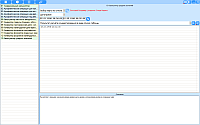Calculator of average values. Calculates average values between two time points (date-time), Julian days and coordinates.
•